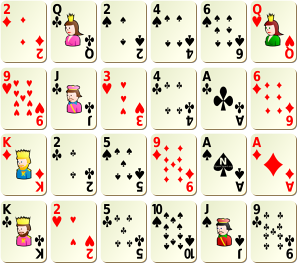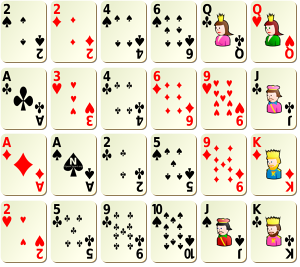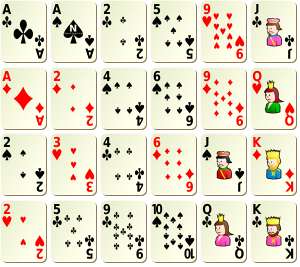Sorting rows and colums in a matrix (with some music, and some magic)

This morning, I was working on some paper on inequality measures, and for computational reasons, I had to sort elements in a matrix. To make it simple, I had a rectangular matrix, like the one below,

> set.seed(1)
> u=sample(1:(nc*nl))
> (M1=matrix(u,nl,nc))
[,1] [,2] [,3] [,4] [,5] [,6]
[1,]    7    5   11   23    6   17
[2,]    9   18    1   21   24   15
[3,]   13   19    3    8   22    2
[4,]   20   12   14   16    4   10

I had to sort elements in this matrix, by row.

> (M2=t(apply(M1,1,sort)))
[,1] [,2] [,3] [,4] [,5] [,6]
[1,]    5    6    7   11   17   23
[2,]    1    9   15   18   21   24
[3,]    2    3    8   13   19   22
[4,]    4   10   12   14   16   20

Nice, elements are sorted by row. But for symmetric reasons, I also wanted to sort them by column. So from this sorted matrix, I decided to sort elements by column,

> (M3=apply(M2,2,sort))
[,1] [,2] [,3] [,4] [,5] [,6]
[1,]    1    3    7   11   16   20
[2,]    2    6    8   13   17   22
[3,]    4    9   12   14   19   23
[4,]    5   10   15   18   21   24

Nice, elements are sorted by column now. Wait… elements are also sorted by row. How comes ? Is it some coincidence ? Actually, no, you can try…

> library(scatterplot3d)
> nc=6; nl=5
> set.seed(1)
> u=sample(1:(nc*nl))
> (M1=matrix(u,nl,nc))
[,1] [,2] [,3] [,4] [,5] [,6]
[1,]    8   23    5   30   10   15
[2,]   11   27    4   29   21   28
[3,]   17   16   13   24   26   12
[4,]   25   14    7   20    1    3
[5,]    6    2   18    9   22   19
> M2=t(apply(M1,1,sort))
> M3=apply(M2,2,sort)
> M3
[,1] [,2] [,3] [,4] [,5] [,6]
[1,]    1    3    7   14   19   22
[2,]    2    6    9   15   20   25
[3,]    4    8   10   17   23   26
[4,]    5   11   16   18   24   29
[5,]   12   13   21   27   28   30

or use the  following function is two random matrices are not enough,

> doublesort=function(seed=2,nl=4,nc=6){
+ set.seed(seed)
+ u=sample(1:(nc*nl))
+ (M1=matrix(u,nl,nc))
+ (M2=t(apply(M1,1,sort)))
+ return(apply(M2,2,sort))
+ }

Please, feel free to play with this function. Because this will always be the case. Of course, this is not a new result. Actually, it is mentioned in More Mathematical Morsels by Ross Honsberger, related to some story on marching band. The idea is simple: consider a marching band, a rectangular one. Here are my players

> library(scatterplot3d)
> scatterplot3d(rep(1:nl,nc),rep(1:nc,each=nl), as.vector(M1),
+ col.axis="blue",angle=40,
+ col.grid="lightblue", main="", xlab="", ylab="", zlab="",
+ pch=21, box=FALSE, cex.symbols=1,type="h",color="red",axis=FALSE)Quite messy, isn’t it ? At least, this is what the leader of the band though, since some tall players were hiding shorter ones. So, he brought the shorter ones forward, and moved the taller ones in the back. But still on the same line,

> m=scatterplot3d(rep(1:nl,nc),rep(1:nc,each=nl), as.vector(M2),
> col.axis="blue",angle=40,
+ col.grid="lightblue", main="", xlab="", ylab="", zlab="",
+ pch=21, box=FALSE, cex.symbols=1,type="h",color="red",axis=FALSE)From the leader’s perspective, everything was fine,

> M=M2
> for(i in 1:nl){
+ for(j in 1:(nc-1)){
+ pts=m\$xyz.convert(x=c(i,i),y=c(j,j+1),z=c(M[i,j],M[i,j+1]))
+ segments(pts\$x,pts\$y,pts\$x,pts\$y)
+ }}But someone in the public (on the right of this graph) did not have the same perspective.

> for(j in 1:nc){
+ for(i in 1:(nl-1)){
+ pts=m\$xyz.convert(x=c(i,i+1),y=c(j,j),z=c(M[i,j],M[i+1,j]))
+ segments(pts\$x,pts\$y,pts\$x,pts\$y)
+ }}So the person in the audience ask – one more time – players to move, but this time, to match with his perspective. Since I consider someone on the right, some minor adjustments should be made here

> sortrev=function(x) sort(x,decreasing=TRUE)
> M3b=apply(M2,2,sortrev)

This time, it is much bettter,

> m=scatterplot3d(rep(1:nl,nc),rep(1:nc,each=nl), as.vector(M3b),
+ col.axis="blue",angle=40,
+ col.grid="lightblue", main="", xlab="", ylab="", zlab="",
+ pch=21, box=FALSE, cex.symbols=1,type="h",color="red",axis=FALSE)And not only from the public’ perspective,

> M=M3b
> for(j in 1:nc){
+ for(i in 1:(nl-1)){
+ pts=m\$xyz.convert(x=c(i,i+1),y=c(j,j),z=c(M[i,j],M[i+1,j]))
+ segments(pts\$x,pts\$y,pts\$x,pts\$y)
+ }}but also for the leader of the marching bandNice, isn’t it ? And why is this property always valid ? Actually, it comes from the pigeonhole theorem (one more time), a nice explanation can be found in The Power of the Pigeonhole by Martin Gardner (a pdf version can also be found on http://www.ualberta.ca/~sgraves/..). As mentioned at the end of the paper, there is also an interpretation of that result that can be related to some magic trick, discussing – in picture – a few month ago on http://www.futilitycloset.com/… : deal cards into any rectangular array:Then put each row into numerical order:Now put each column into numerical order:That last step hasn’t disturbed the preceding one: rows are still in order. And that’s a direct result from  pigeonhole theorem. That’s awesome, isn’t it ?

Sur le lissage exponentiel

Sur le lissage exponentiel, je pourrais renvoyer vers Gardner (1985), et la version remise au goût du jour, Gardner (2005). J’avais pris comme position, dans le cours, de présenter rapidement le lissage exponentiel, en notant qu’on les évoquerais à nouveau plus tard comme cas particulier des modèles ARIMA. Comme le note la dernière version, Gardner (2005), ce n’est pas forcément l’unique manière de voir, et le cours pourrait être orienté complètement autour des notions de lissage exponentiel (c’est d’ailleurs le point du vue de livre Hyndman, Koehler, Ord & Snyder (2008)), “when Gardner (2005) appeared, many believed that exponential smoothing should be disregarded because it was either a special case of ARIMA modeling or an ad hoc procedure with no statistical rationale. As McKenzie (1985) observed, this opinion was expressed in numerous references to my paper. Since 1985, the special case argument has been turned on its head, and today we know that exponential smoothing methods are optimal for a very general class of state-space models that is in fact broader than the ARIMA class.”

N’étant toujours pas convaincu, j’évoquerais à nouveau le lissage exponentiel quand on présentera les modèles ARIMA. En attendant, un peu de code pour mieux comprendre ce qui est fait quand on fait du lissage exponentiel.

Commençons par le lissage exponentiel simple, i.e.désigne un poids attribué à la nouvelle observation dans la fonction de lissage. Le code pour lisser une série est alors assez simple,

> library(datasets)
> X=as.numeric(Nile)
> Lissage=function(a){
+  T=length(X)
+  L=rep(NA,T)
+  L=X
+  for(t in 2:T){L[t]=a*X[t]+(1-a)*L[t-1]}
+  return(L)
+ }
> plot(X,type="b",cex=.6)
> lines(Lissage(.2),col="red")Sur la figure ci-dessus, non visualise l’impact desur le lissage. La prévision que l’on fait à la date, pour un horizonest alors. Il est alors possible de voir le poids comme un paramètre et on va alors chercher le poids optimal. La stratégie classique est de minimiser l’erreur de prédiction commise à un horizon de 1> V=function(a){
+  T=length(X)
+  L=erreur=rep(NA,T)
+  erreur=0
+  L=X
+  for(t in 2:T){
+  L[t]=a*X[t]+(1-a)*L[t-1]
+  erreur[t]=X[t]-L[t-1] }
+ return(sum(erreur^2))
+ }

Ici, on obtient comme poids optimal

> A=seq(0,1,by=.02)
> Ax=Vectorize(V)(A)
> plot(A,Ax,ylim=c(min(Ax),min(Ax)*1.05))
> optimize(V,c(0,.5))\$minimum
 0.246581On notera que c’est ce que suggère la fonction de R,

> hw=HoltWinters(X,beta=FALSE,gamma=FALSE,l.start=X)
> hw
Holt-Winters exponential smoothing without trend an seasonal comp.

Call:
HoltWinters(x = X, beta = FALSE, gamma = FALSE, l.start = X)

Smoothing parameters:
alpha:  0.2465579
beta :  FALSE
gamma:  FALSE

Coefficients:
[,1]
a 805.0389

> plot(hw)
> points(2:(length(X)+1),Vectorize(Lissage)(.2465),col="blue")Dans le cas du lissage exponentiel double, i.e.etDans ce cas, la prédiction est. Le code pour faire un lissage double est là encore assez simple,

> Lissage=function(a,b){
+  T=length(X)
+  L=B=rep(NA,T)
+  L=X; B=0
+  for(t in 2:T){
+  L[t]=a*X[t]+(1-a)*(L[t-1]+B[t-1])
+  B[t]=b*(L[t]-L[t-1])+(1-b)*B[t-1] }
+ return(L)
+ }

Sur la figure suivante, on visualise l’évolution du lissage en fonction de(étant ici fixé),(le lissage simple – avec le même poids– apparaissant ici en trait clair).